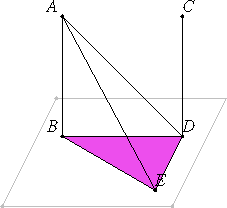# Proposition 8

If two straight lines are parallel, and one of them is at right angles to any plane, then the remaining one is also at right angles to the same plane.

Let AB and CD be two parallel straight lines, and let one of them, AB, be at right angles to the plane of reference.

I say that the remaining one, CD, is also at right angles to the same plane.XI.7

Let AB and CD meet the plane of reference at the points B and D. Join BD. Then AB, CD, and BD lie in one plane.

Draw DE in the plane of reference at right angles to BD, make DE equal to AB, and join BE, AE, and AD

XI.Def.3

Now, since AB is at right angles to the plane of reference, therefore AB is also at right angles to all the straight lines which meet it and lie in the plane of reference. Therefore each of the angles ABD and ABE is right.

And, since the straight line BD falls on the parallels AB and CD, therefore the sum of the angles ABD and CDB equals two right angles.

I.29

But the angle ABD is right, therefore the angle CDB is also right. Therefore CD is at right angles to BC.

I.4

And since AB equals DE, and BD is common, the two sides AB and BD equal the two sides ED and DB, and the angle ABD equals the angle EDB, for each is right, therefore the base AD equals the base BE.

I.8

And since AB equals DE, and BE equals AD, the two sides AB and BE equal the two sides ED and DA respectively, and AE is their common base, therefore the angle ABE equals the angle EDA.

XI.4

But the angle ABE is right, therefore the angle EDA is also right. Therefore ED is at right angles to AD. But it is also at right angles to DB. Therefore ED is also at right angles to the plane through BD and DA.

Therefore ED also makes right angles with all the straight lines which meet it and lie in the plane through BD and DA. But DC lies in the plane through BD and DA inasmuch as AB and BD lie in the plane through BD and DA, and DC also lies in the plane in which AB and BD lie.

XI.4

Therefore ED is at right angles to DC, so that CD is also at right angles to DE. But CD is also at right angles to BD. Therefore CD is set up at right angles to the two straight lines DE and DB so that CD is also at right angles to the plane through DE and DB.

But the plane through DE and DB is the plane of reference, therefore CD is at right angles to the plane of reference.

Therefore, if two straight lines are parallel, and one of them is at right angles to any plane, then the remaining one is also at right angles to the same plane.

Q.E.D.

## Guide

This is a converse of proposition XI.6.

This proposition is used in the proof of the next one as well as several others in this book.